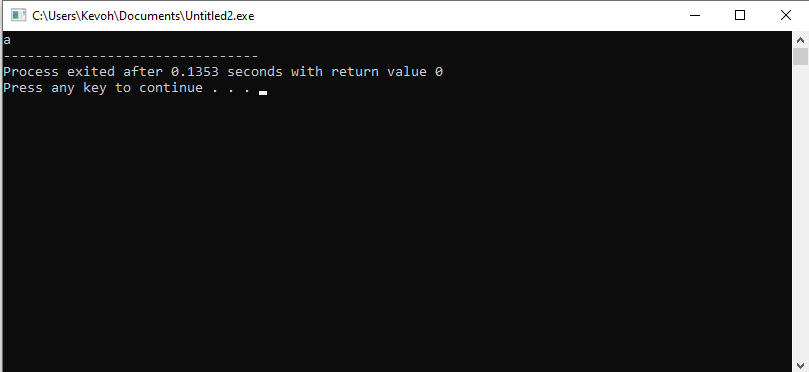# How to convert int to char C++

Published July 26, 2022

There are various situations where we need to convert an int into a char, especially when you would like to declare a character variable type or simply want to store only a single character. In this article, we are going to learn how you can convert an int into a char in C++.

Let's get started.

## How to convert an int into a Char in C++

While this seems like a difficult task, especially for C++ beginners, converting an int into a char in C++ is pretty simple. Just follow the simple steps of Typecasting method:

Step 1: Declaration and initialization of the variables. In this case, we will initialize our integer value. This is the integer we are going to convert into a character.

Step 2: Typecast- in this step, we are going to transform one data type into another. In this case, we are going to typecast integer a and save the value in the char data type.

Step 3: Print out the Char. Now output your character using the cout.

The following example shows an example of the conversion of an int into a char in C++

## Example

 #include using namespace std; int main() { int a = 97; cout << char(a); return 0; }

OutputArticle Contributed By :217 Views
Projects By category
Categories